# 6-Card Poker Hands

Brian Alspach

17 January 2000

### Abstract:

We determine the number of 6-card poker hands.

There are a few 6-card poker games so it is worth looking at probabilities for winning with certain kinds of hands. One chooses the highest ranked 5-card poker hand among the 6 cards and values the hand based on the 5-card hand. The types of 5-card poker hands in decreasing rank are

• straight flush
• 4-of-a-kind
• full house
• flush
• straight
• 3-of-a-kind
• two pairs
• a pair
• high card

The total number of 6-card poker hands is.

A straight flush is completely determined once the smallest card in the straight flush is known. There are 40 cards eligible to be the smallest card in a straight flush. If the smallest card in the straight flush is an ace, then the sixth card may be any of 47 cards. If the smallest card in the straight flush is any of the other 36 eligible beginning cards, then the sixth card may be any of 46 cards because we cannot use the next smallest card in the same suit as the straight flush. Hence, there are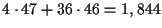6-card hands containing straight flushes.

In forming a 4-of-a-kind hand, there are 13 choices for the rank of the quads, 1 choice for the 4 cards of the given rank, and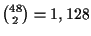choices for the remaining 2 cards. This implies there are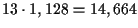4-of-a-kind hands.

There are 2 ways to get a full house and we count them separately. One way of obtaining a full house is for the 6-card hand to contain 2 sets of triples. There are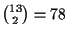ways to choose the 2 ranks and 4 ways to choose each of the triples. This gives us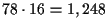full houses of this type. The other way of getting a full house is for the 6-card hand to contain a triple, a pair and a remaining card of a third rank. There are 13 choices for the rank of the triple, 12 choices for the rank of the pair, and 44 choices for the singleton card. There are 4 ways of choosing the triple of a given rank and 6 ways to choose the pair of the other rank. This produces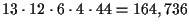full houses of the latter type. Adding the two numbers yields 165,984 full houses.

To count the number of flushes, we obtain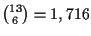6-card hands formed from cards in the same suit. Altogether, there are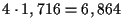flushes with 6 cards in the same suit. There are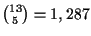choices for 5 cards in the same suit. There are then 39 choices for a sixth card from a different suit. Thus, there are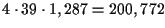flushes in 6-card hands, where precisely 5 cards are in the same suit. Combining the two gives us 207,636 6-card hands containing flushes. Of these, 1,844 are straight flushes whose removal leaves 205,792 flushes.

Let's determine how many sets of 6 distinct ranks correspond to straights. One possible form is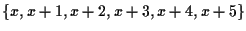, where x can be any of 9 ranks. The other possible form is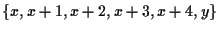, where yis neither x-1 nor x+5. When x is ace or 10, then there are 7 choices for y. When x is between 2 and 9, inclusive, there are 6 choices for y. This implies there are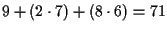sets of 6 distinct ranks corresponding to straights. There are then 4 choices for each card of the given ranks except we must remove those choices producing flushes. There are 4 choices giving all 6 cards in the same suit. If 5 are in the same suit, there are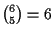choices of which 5 ranks will be in the same suit, 4 choices for the suit of the 5 cards, and 3 choices for the suit of the remaining card. So there are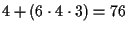choices which give a flush. This means there are 46 - 76 = 4,020 choices not producing a flush. Hence, there are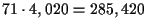straights of this form.

We also can have a set of 5 distinct ranks producing a straight which means the corresponding 6-card hand must contain a pair as well. There are 10 sets of ranks of the form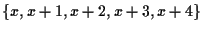. There are 5 choices of the rank to be paired, 6 choices for the pair, and 4 choices for each of the other 4 cards except not all 4 cards can be chosen in the same suit as either of the cards in the pair. This means there are 44 - 2 = 254 choices for the 4 cards. We then have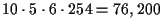straights of this form. Altogether there are 361,620 straights.

In forming a 3-of-a-kind hand, there must be a triple and 3 other cards all of distinct ranks different from the rank of the triple. There are 13 choices for the rank of the triple, and there are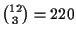choices for the ranks of the other 3 cards. There are 4 choices for the triple of the given rank and there are 4 choices for each of the cards of the remaining 3 ranks. Altogether, we have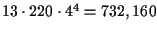3-of-a-kind hands.

Next we consider two pairs hands. Such a hand may contain either three pairs, or two pairs plus two remaining cards of distinct ranks. We evaluate these 2 types of hands separately. If the hand has three pairs, there are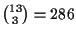ways to choose the ranks of the pairs and 6 ways to choose each of the pairs. This produces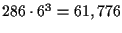6-card hands with three pairs.

For the other kind of two pairs hand, there arechoices for the two ranks of the pairs and there are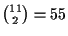ways to choose the ranks of the 2 singletons. There are 6 choices for each of the pairs, and there are 4 choices for each of the remaining 2 cards. This produceshands of two pairs of the second type. Adding the two gives 2,532,816 6-card hands with two pairs.

Now we count the number of hands with a pair. Such a hand must have 5 distinct ranks. There arepossible sets of 5 ranks. We must remove sets of the formbecause these correspond to straights. There are 10 such sets leaving 1,277 sets of ranks corresponding to a hand with one pair. Given such a set, there are 5 choices for the rank of the pair, and 6 choices for a pair of the chosen rank. There are 4 choices for each of the remaining 4 cards except we cannot choose all 4 to be in the same suit as either of the cards forming the pair. Hence, there are 44-2 = 254 choices for the remaining 4 cards. This gives us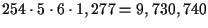hands with a pair.

We could determine the number of high card hands by removing the hands which have already been counted in one of the previous categories. Instead, let us count them independently and see if the numbers sum to 20,358,520 which will serve as a check on our arithmetic.

A high card hand has 6 distinct ranks, but does not include straights. So we must eliminate sets of ranks which have 5 consecutive ranks. In determining the number of straights above, we derived that there are 71 sets of 6 distinct ranks which give straights. There aresets of 6 distinct ranks. Removing the 71 sets corresponding to straights, leaves 1,645 sets of distinct ranks which do not produce straights. There are 4 choices for each of the 6 cards in a given set producing 46 = 4,096 ways of choosing cards for a given set of ranks. However, some of the choices produce flushes and we must remove them. Clearly there are 4 ways of choosing the 6 cards all in the same suit which is one way of getting a flush. There 6 ways of choosing 5 of the ranks and 4 choices for the suit of these 5 ranks, and 3 choices for the suit of the remaining card. This gives us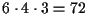choices of suits which produce a flush with 5 cards in the same suit. We remove these 76 choices which produce flushes giving us 4,020 choices for the 6 cards which do not produce a flush. Multiplying 4,020 by 1,645 gives 6,612,900 high card hands.

If we sum the preceding numbers, we obtain 20,358,520 and we can be confident the numbers are correct.

Here is a table summarizing the number of 6-card poker hands. The probability is the probability of having the hand dealt to you when dealt 6 cards.

 hand number Probability straight flush 1,844 .000091 4-of-a-kind 14,664 .00072 full house 165,984 .00815 flush 205,792 .0101 straight 361,620 .0178 3-of-a-kind 732,160 .036 two pairs 2,532,816 .1244 pair 9,730,740 .478 high card 6,612,900 .325

You will observe that you are less likely to be dealt a hand with no pair (or better) than to be dealt a hand with one pair. This has caused some people to query the ranking of these two hands. In fact, if you were ranking 6-card hands based on 6 cards, the order of the last 2 would switch. However, you are basing the ranking on 5 cards so that if you were to rank a high card hand higher than a hand with a single pair, people would choose to ignore the pair in a 6-card hand with a single pair and call it a high card hand. This would have the effect of creating the following distortion. There are 16,343,640 6-card hands containing 5 cards which are high card hands. Of these 16,343,640 hands, 9,730,740 also contain 5-card hands which have a pair. Thus, the latter hands are more special and should be ranked higher (as they indeed are) but would not be under the scheme being discussed in this paragraph.

Home | Publications | Preprints | MITACS | Poker Digest | Graph Theory Workshop | Poker Computations | Feedback
website by the Centre for Systems Science
last updated 12 January 2000